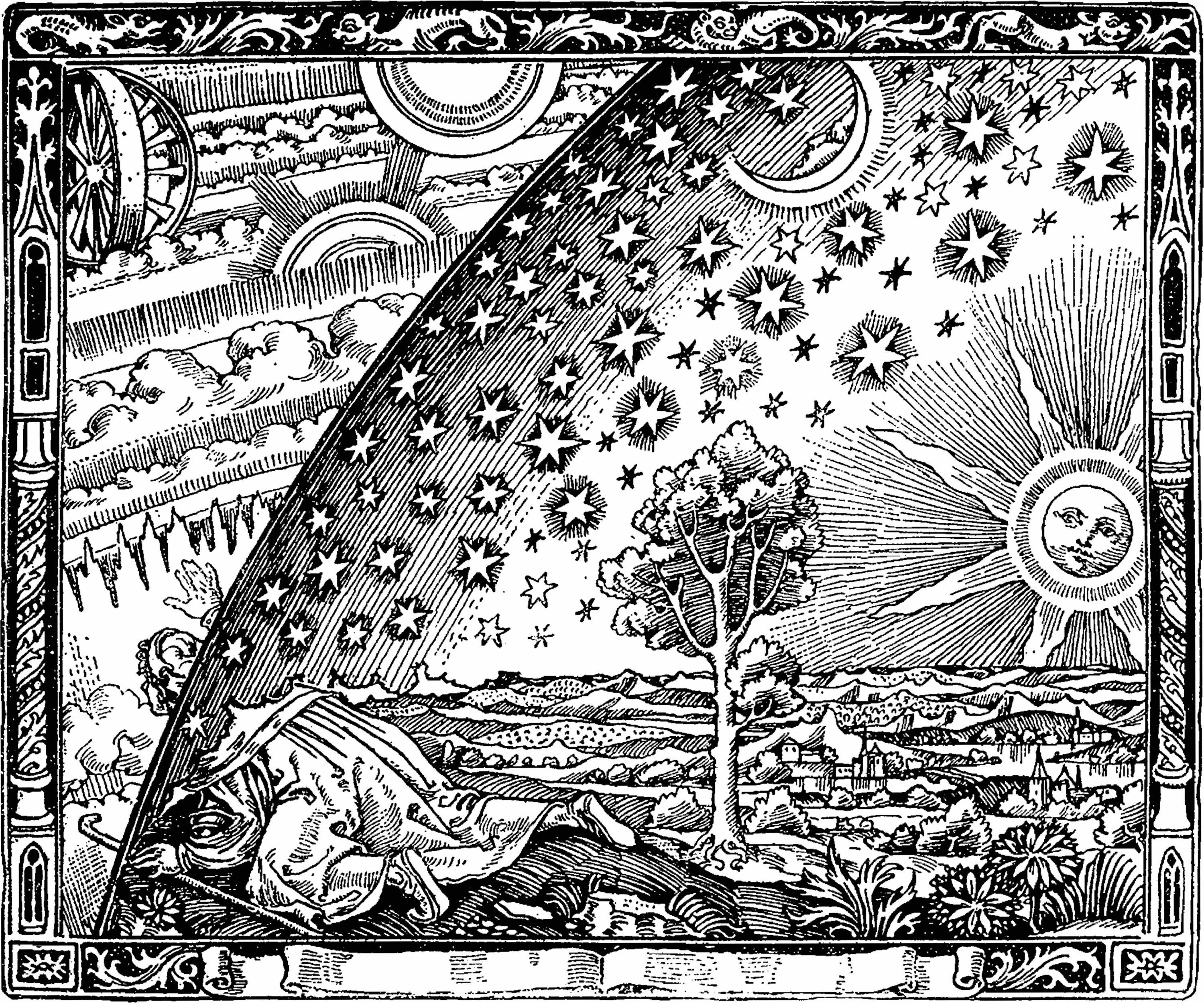# MATHEMATICAL METHODS I

PHYS 508, Fall 2019

9:30 to 10:50 Monday, Wednesday, Room 136 Loomis Laboratory.

Intructor: Professor Michael Stone

This web-page contains links to documents such as handouts and other useful stuff. These files are now only in PDF (.pdf) format.

Syllabus Outline

The course covers four related areas:

• Calculus of Variations. Equations of mathematical physics as variational problems, conservation laws, Lagrange multipliers, origin of eigenproblems, variational approximation schemes.
• Ordinary differential equations. Linear equations: Solution space, linear independence, Wronskians, normal forms. Eigenvalue problems: importance of boundary conditions, formal and true self-adjointness, completeness of eigenfunctions, Fourier series, continuous spectra and Fourier integrals. Green Functions: Range-nullspace theorem, Fredholm alternative, constructing Green functions via jump conditions.
• Partial Differential equations. Classification of PDE's. Hyperbolic equations: wave equation, method of characteristics, shocks and weak solutions. Heat equation: solution by integral transforms. Elliptic equations: Dirichlet and Neumann problems, Poisson's equation, Legendre functions, spherical harmonics, Bessel and spherical Bessel functions, examples from electrostatics.
• Integral Equations. Type I and type II Fredholm and Volterra equations, solution via Fourier and Laplace transforms, Abel's equation. Separable Kernels: compact and Hilbert-Schmidt operators, Fredholm alternative again. Perturbation methods: Neumann and Fredholm series.

Homework Sets

There are 11 weekly homework sets that will count towards the final grade, and one end-of-term set of optional problems. Your solutions sets must be deposited in the Phys 508 homework box before 5pm on the due date, which will usually be a Monday. Exams

The midterm will be in class on November 6th. There will be review session in class on Monday 4th. The exam will cover everything up to the end of chapter 5.

The final exam will be in our usual classroom on Wednesday Dec 18th from 1:30 to 4:30pm. It will cover everything.

Some old exams for you to review:

Midterm Exam, Fall 2013

Final Exam, Fall 2003

Final Exam, Fall 2014

Textbook

I recommend (but do not require) that you purchase Mathematics for Physics: A guided tour for graduate students by myself and Paul Goldbart. (Cambridge University Press 2009). The list price is \$90, but Amazon has it for \$72 (+shipping). I do not yet know what the UI bookstore is selling it for. (I do not recommend the Kindle version of the Book. The equations do not scale correctly.) Our book is an expanded version of the lecture notes for both PHYS 508 and PHYS 509. Here is a list of typos and outright errors that readers have found in the printed text.

A draft version of the book is still available for download, but, now that it is published, I am no longer maintaining this version, so typos are not being corrected.

As a cheaper alternative you can buy Mathematics for Physicists by Phillipe Dennery and Andre Krzywicki (Dover Publications, \$12.95). This book covers a fair bit of the material in this course, and will be useful for the complex-variable part of PHYS 509.

Your grade in the course will be determined as from your total scores weighted as follows: Homework 50%, Midterm exam 20%, Final Exam 30%.

Some of the material in the course is supposed to introduce you to the wider culture of mathematical physics and its applications in the real world. Here are links relating to some of the topics discussed:
• People A Short Biography of George Green can be found here. This site also contains the biographies of many other mathematicians and mathematical physicists.
• Wave Phenomena A video of a tidal bore can be found here here. Images and description of the Kelvin ship wake can be found here and here
• Solitons A nonlinear pulse obeying the KdV equation does not form a shock, but instead decays into solitions. Here is a movie of this phenomenon. It is taken from the ``soliton lab'' created by Kanehisa Takasaki at the univerity of Kyoto.

Staff

Finding me:
Office: 2117 ESB.
Phone: 3-2891.
e-mail: m-stone5@illinois.edu
My office hour is Moday 8-9am, outside 2117 ESB. If this is inconvenient for you send me an email and I will schedule an appointment at another time.